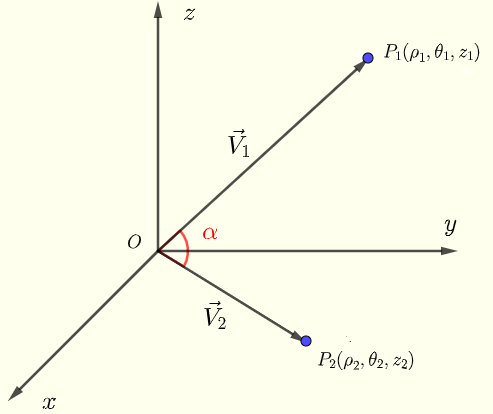# Angle Between two Vectors in Cylindrical Coordinates - Calculator

## Formulas Used in Calculations

An online calculator to calculate angle $\alpha$ between these two vectors by their cylindrical coordinates is presented
Given two vectors whose initial point is the origin of a system of cylindrical coordinates and terminal points $P_1(\rho_1,\theta_1,z_1)$ and $P_2(\rho_2,\theta_2,z_2)$ given by their cylindrical coordinates.Fig.1 - Angle $\alpha$ between two vetcors
Convert the cylindrical coordinates of points $P_1(\rho_1,\theta_1,z_1)$ and point $P_2(\rho_2,\theta_2,z_2)$ into rectangular coordinates $P_1(x_1,y_1,z_1)$ and $P_2(x_2,y_2,z_2)$ where
$x_1 = \rho_1 \cos \theta_1$ , $y_1 = \rho_1 \sin \theta_1$ , $z_1 = z_1$
$x_2 = \rho_2 \cos \theta_2$ , $y_2 = \rho_2 \sin \theta_2$ , $z_2 = z_2$

The vectors $\; \vec{OP_1} = \vec V_1$ and $\; \vec{OP_2} = \vec V_2$ have the components
$\vec V_1 \lt x_1 , y_1 , z_1 \gt$ and $\; \vec V_2 \lt x_2 , y_2 , z_2 \gt$

The dot product of $\vec V_1$ and $\vec V_2$ is given by
$\vec V_1 \cdot \vec V_2 = ||\vec V_1 || \cdot ||\vec V_1 || \cos \alpha$
Hence
$\alpha = \arccos \left(\dfrac {\vec V_1 \cdot \vec V_2}{||\vec V_1 || \cdot ||\vec V_1 ||} \right)$
where
$\vec V_1 \cdot \vec V_2 = x_1 x_2 + y_1 y_2 + z_1 z_2$
and
$||\vec V_1 || = \sqrt {x_1^2 + y_1^2 + z_1^2}$ and $||\vec V_2 || = \sqrt {x_2^2 + y_2^2 + z_2^2}$

Note that if $||\vec V_1 || = 0$ or $||\vec V_2 || = 0$, the angle between the two vectors is undefined

## Use Calculator to Calculate Angle Bewteen two Vectors in Cylindrical Coordinates

1 - Enter the cylindrical coordinates $\rho_1$ , $\theta_1$, $z_1$ of point $P_1$, and $\rho_2$ , $\theta_2$, $z_2$ of point $P_2$, selecting the desired units for the angles, and press the button "Calculate". You may also change the number of decimal places as needed; it has to be a positive integer.

 $\rho_1 =$ 1 $\theta1 =$ 45 degrees radians $z_1 =$ 45 $\rho_2 =$ 2 $\theta2 =$ 90 degrees radians $z_2 =$ 30
Number of Decimal Places =

$\alpha =$ (degrees)
$\alpha =$ (radians)